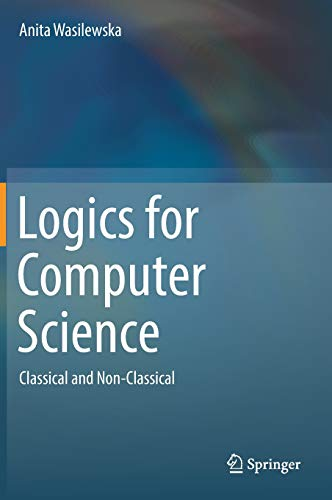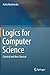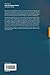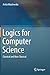Thursday , February 20th 2020

# Logics for Computer Science: Classical and Non-Classical#### Product Features:

Providing an in-depth introduction to fundamental classical and non-classical logics, this textbook offers a comprehensive survey of logics for computer scientists. Logics for Computer Science contains intuitive introductory chapters  explaining the  need for logical investigations, motivations for different types of logics  and some of their history. They are followed by strict …
Price as on: 2020-01-30 16:49:59

#### Product Description

Providing an in-depth introduction to fundamental classical and non-classical logics, this textbook offers a comprehensive survey of logics for computer scientists. Logics for Computer Science contains intuitive introductory chapters  explaining the  need for logical investigations, motivations for different types of logics  and some of their history. They are followed by strict  formal approach chapters. All chapters contain many detailed examples explaining each of the introduced notions and definitions, well chosen sets of exercises with carefully written solutions, and sets of homework.
While many logic books are available, they were written by logicians for  logicians, not for computer scientists. They usually choose one particular way of presenting the material and use a specialized language. Logics for Computer Science discusses Gentzen as well as Hilbert formalizations, first order theories, the Hilbert Program, Godel’s  first and second incompleteness theorems and their proofs. It also introduces and discusses some many valued logics, modal logics and introduces algebraic models  for classical, intuitionistic, and modal S4 and  S5 logics.

The theory of computation is based on concepts defined by logicians and mathematicians. Logic plays a fundamental role in computer science, and this book explains the basic theorems, as well as different techniques of proving them in classical and some non-classical logics. Important applications derived from concepts of logic for computer technology include Artificial Intelligence and Software Engineering. In addition to Computer Science, this book may also find an audience in mathematics and philosophy courses, and some of the chapters are also useful for a course in Artificial Intelligence.

## The Design and Analysis of Computer

With this text, you gain an understanding of the fundamental concepts of algorithms, the very heart of computer science.…

## Mathematics for 3D Game Programming and Computer

This updated third edition illustrates the mathematical concepts that a game developer needs to develop 3D computer graphics and…